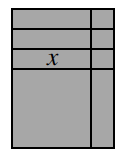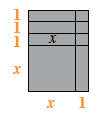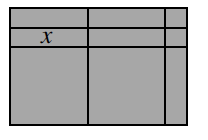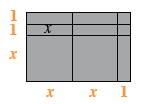### Home > CAAC > Chapter 5 > Lesson 5.1.2 > Problem5-15

5-15.

Examine the rectangles formed with tiles below. For each figure, write its area as a product of the width and length and as a sum of its parts.

a.• Find the outside dimensions of the large rectangle.

See dimensions below.As a product: multiply the dimensions of the large rectangle together.
As a sum: add up the areas of all of the small rectangles.

area as product $=$ (length)(width) $=(x+3)(x+1)$
area as sum $=x+x+x+x^2+1+1+1+x=x^2+4x+3$

1.• Use the same method as part (a).area as a product $=$ (length)(width) $=(2x+1)(x+2)$
product as a sum $=x^2+x^2+x+x+x+x+x+1+1=2x^2+5x+2$